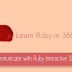## Ruby Tutorial for Beginners : IRB

What is a variable ?

A variable is a piece of data that point to a specific name. For instance x=a+b where a and b can take many different values.

Open up command prompt (Ctrl+Alt+T or Win+R "Cmd")

\$ irb

irb(main):001:0> a=15
=> 15
irb(main):002:0> b=52
=> 52
irb(main):003:0> c=a+b
=> 67
irb(main):004:0>

If you observe the above snippet, a and b store 15, 52 respectively. c stores the summation of a and b.

Float Type

In Ruby, floats can be defined either with numbers containing a decimal place.

irb(main):001:0> x=3.03
=> 3.03
irb(main):002:0> y=6.3
=> 6.3
irb(main):003:0> z=x+y
=> 9.33
irb(main):004:0> z
=> 9.33
irb(main):005:0>

Strings

Strings have one or more characters surrounded by quotes. For instance x="Welcome to Ruby programming"

irb(main):001:0> x="Hello"
=> "Hello"
irb(main):002:0> y=",World"
=> ",World"
irb(main):003:0> x
=> "Hello"
irb(main):004:0> y
=> ",World"
irb(main):005:0> d=x+"Hello"
=> "HelloHello"
irb(main):006:0> e=x+y+"Nice"
=> "Hello,WorldNice"
irb(main):007:0> e
=> "Hello,WorldNice"
irb(main):008:0>

x contains a string "Hello", y also contains a string ",World". We can concatenate two or more strings using '+' operator.
e=x+y+"Nice"

Boolean

Booleans can hold only two values true or false.

irb(main):001:0> x=false
=> false
irb(main):002:0> x
=> false
irb(main):003:0>

Next lesson we will learn some arithmetical and mathematical operations.
Ruby Tutorial : Let's communicate with Ruby Interactive Shell (IRB)Reviewed by Nikin on 10 July Rating: 5